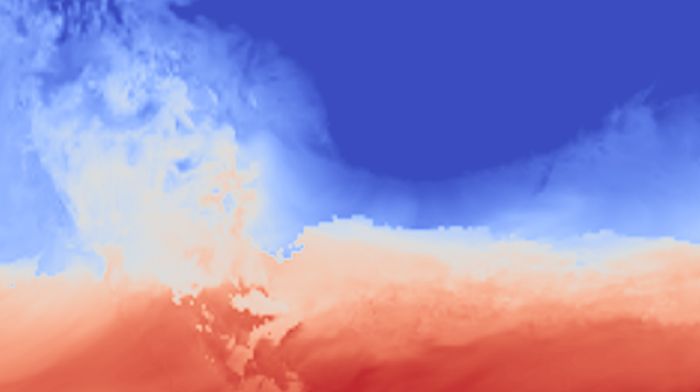# Ryoko Araki# 4 ways to find out Coordinate Reference System information (CRS) and set it in a xarray

When you want to process raster data (arrays with geo-coordinates) with Python, there are several options. It was the most straightforward for me to use Python, read all the data in xarray and process it with rasterio using rioxarray (tutorial by University of Colorado Boulder here).

Rioxarray requires data arrays, x-y coordinate array, and coordinate reference system information (CRS) to function. However, it is not always easy to find out and set the CRS, as different files stores CRS in different places (I wish for more advancement of data-standardization!).

In this article, I summarize 4 ways to find out and set the CRS in a xarray dataset.
Disclaimer: I am not the super expert on the projection system, so please use the code at your own risk and let me know when you find any mistakes!

To start off, I import libraries as follows.

``````# Import library
import rioxarray as rio
import xarray as xr
from osgeo import gdal
from pyproj import CRS``````
Python

## Method 1. Get CRS info with gdal (the easiest option)

If the data is well-prepared for geo-processing, you will not need to dig into the data file. Gdal will do the job.

``````# Open data with Gdal and get the CRS information
ds0 = gdal.Open(file_path)
crs = CRS.from_string(ds0.GetProjection())

# Open data with xarray and set the CRS information obtained above
ds = xr.open_dataset(file_path)
ds.rio.write_crs(crs.to_string(), inplace = True)``````
Python

## Method 2. Read a wkt or proj4 string from a file

If the Method 1 does not work, you have to dig into the data file and find out where the CRS information is located.

``````# Open data with xarray
ds = xr.open_dataset(file_path)

# Locate CRS info in the file manually
cc = ds.TEMP.attrs.crs

# Set the CRS information obtained above
ds.rio.write_crs(cc.to_string(), inplace = True)``````
Python

## Method 3. Read CF from a file, and convert it to CRS

Similar to the second method. However, sometimes in the NetCDF file, the coordinate information is stored in the CF convention, which need conversion to CRS (details in pyproj website).

``````# Open data with xarray
ds = xr.open_dataset(file_path)

# Locate CRS info in CF convention in the variable file, convert it to CRS format, and read the string as cc
cc = CRS.from_cf(ds.crs.attrs)

# Set the CRS information obtained above
ds.rio.write_crs(cc.to_string(), inplace = True)``````
Python

## Method 4. Write a wkt or proj4 string

Last, if the above all did not work, you can create a CRS string by yourself. Find out where the projection, the parameters for the projection, the datum, and the spheroid are located.

Here are some useful links for this method.
To find out the compatibility of different parameter names for common projection: https://cfconventions.org/wkt-proj-4.html
To find out the formatting template for wkt or proj4 strings (you will only need to tweak the parameters): http://epsg.io/4326

``````# Open data with xarray
ds = xr.open_dataset(file_path)

# Create a CRS string
crs =
'GEOGCS["HRAP_Sphere",DATUM["",SPHEROID["",6371200.0,0.0],PRIMEM["Greenwich",0.0], PARAMETER["false_easting",1905000],PARAMETER["false_northing",7620000], PARAMETER["latitude_of_origin",60], PARAMETER["longitude_of_origin",-105], UNIT["Degree",0.0174532925199433]]'

# Set the CRS information obtained above
ds.rio.write_crs(crs, inplace=True)``````
Python

By the way, specifically for the WRF-Hydro grid, I wrote as follows (referring to this blogpost). WRF-Hydro is national hydrologic model in the U.S., and it uses their own grid definitions.

``````# Open data with xarray
ds0 = xr.open_dataset(file_path)

# Create a CRS string
crs = '+proj=lcc +lat_1=39 +lat_2=50 +lat_0=39 +lon_0=-135 +a=6370 +b=6370 +towgs84=0,0,0 +no_defs'

# Set the CRS information obtained above
ds0.rio.write_crs(crs1, inplace=True)

# Re-create an xarray object
ds = xr.DataArray(
data= ds0.values,
dims=['y', 'x'],
coords={
'y': ds0.y.values,
'x': ds0.x.values
},
attrs={'_FillValue': np.nan}
)

# Re-project the xarray to WGS1984
ds.rio.write_crs("EPSG:4326", inplace=True)<br><br><br>``````
Python

Hope it helps!!